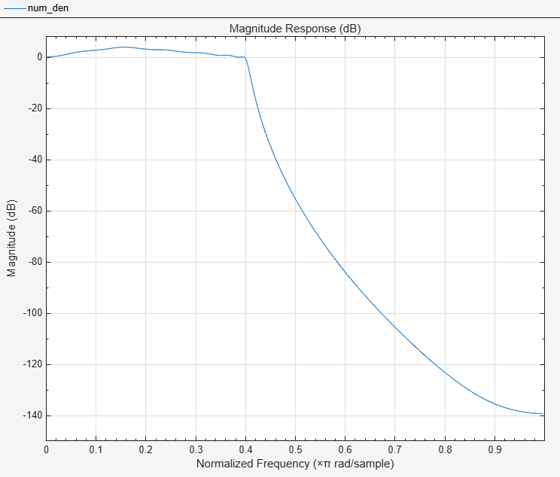# iirlpnorm

Least P-norm optimal IIR filter

## Syntax

```[num,den] = iirlpnorm(n,d,f,edges,a) [num,den] = iirlpnorm(n,d,f,edges,a,w) [num,den] = iirlpnorm(n,d,f,edges,a,w,p) [num,den] = iirlpnorm(n,d,f,edges,a,w,p,dens) [num,den] = iirlpnorm(n,d,f,edges,a,w,p,dens,initnum,initden) [num,den,err] = iirlpnorm(...) [num,den,err,sos,g] = iirlpnorm(...) ```

## Description

`[num,den] = iirlpnorm(n,d,f,edges,a)` returns a filter having a numerator order `n` and denominator order `d` which is the best approximation to the desired frequency response described by `f` and `a` in the least-pth sense. The vector `edges` specifies the band-edge frequencies for multi-band designs. An unconstrained quasi-Newton algorithm is employed and any poles or zeros that lie outside of the unit circle are reflected back inside. `n` and `d` should be chosen so that the zeros and poles are used effectively. See the Hints section. Always use `freqz` to check the resulting filter.

`[num,den] = iirlpnorm(n,d,f,edges,a,w)` uses the weights in `w` to weight the error. `w` has one entry per frequency point (the same length as `f` and `a`) which tells `iirlpnorm` how much emphasis to put on minimizing the error in the vicinity of each frequency point relative to the other points. `f` and `a` must have the same number of elements, which may exceed the number of elements in `edges`. This allows for the specification of filters having any gain contour within each band. The frequencies specified in `edges` must also appear in the vector `f`. For example,

```[num,den] = iirlpnorm(5,12,[0 .15 .4 .5 1],[0 .4 .5 1],... [1 1.6 1 0 0],[1 1 1 10 10])```

is a lowpass filter with a peak of 1.6 within the passband.

`[num,den] = iirlpnorm(n,d,f,edges,a,w,p)` where `p` is a two-element vector [`pmin pmax`] allows for the specification of the minimum and maximum values of `p` used in the least-pth algorithm. Default is [2 128] which essentially yields the L-infinity, or Chebyshev, norm. `Pmin` and `pmax` should be even. If `p` is `'inspect'`, no optimization will occur. This can be used to inspect the initial pole/zero placement.

`[num,den] = iirlpnorm(n,d,f,edges,a,w,p,dens)` specifies the grid density `dens` used in the optimization. The number of grid points is `(dens*(n+d+1))`. The default is 20. `dens` can be specified as a single-element cell array. The grid is not equally spaced.

`[num,den] = iirlpnorm(n,d,f,edges,a,w,p,dens,initnum,initden)` allows for the specification of the initial estimate of the filter numerator and denominator coefficients in vectors `initnum` and `initden`. `initnum` should be of length n+1, and `initden` should be of length d+1. This may be useful for difficult optimization problems. The pole-zero editor in Signal Processing Toolbox™ software can be used for generating `initnum` and `initden`.

`[num,den,err] = iirlpnorm(...)` returns the least-pth approximation error, `err`.

`[num,den,err,sos,g] = iirlpnorm(...)` returns the second-order section representation in the matrix `sos` and gain `g`. For numerical reasons it may be beneficial to use `sos` and `g` in some cases.

## Examples

Least Pth-Norm Optimal IIR Filter Design

### Design a Lowpass Filter with a Peak of 4.015 dB in Passband

```[num,den] = iirlpnorm(5,12,[0 .15 .4 .5 1],[0 .4 .5 1],... [1 1.6 1 0 0],[1 1 1 10 10])```
```num = 1×6 0.0028 0.0036 0.0018 0.0005 0.0001 0.0000 ```
```den = 1×13 1.0000 -6.6498 22.6915 -51.5423 85.9098 -109.9888 110.4802 -87.5412 54.2750 -25.7000 8.8451 -1.9963 0.2255 ```

Display the magnitude response in fvtool

`fvtool(num,den)`## Hints

• This is a weighted least-pth optimization.

• Check the radii and locations of the poles and zeros for your filter. If the zeros are on the unit circle and the poles are well inside the unit circle, try increasing the order of the numerator or reducing the error weighting in the stopband.

• Similarly, if several poles have a large radii and the zeros are well inside of the unit circle, try increasing the order of the denominator or reducing the error weighting in the passband.

## References

Antoniou, A., Digital Filters: Analysis, Design, and Applications, Second Edition, McGraw-Hill, Inc. 1993.

## Extended Capabilities